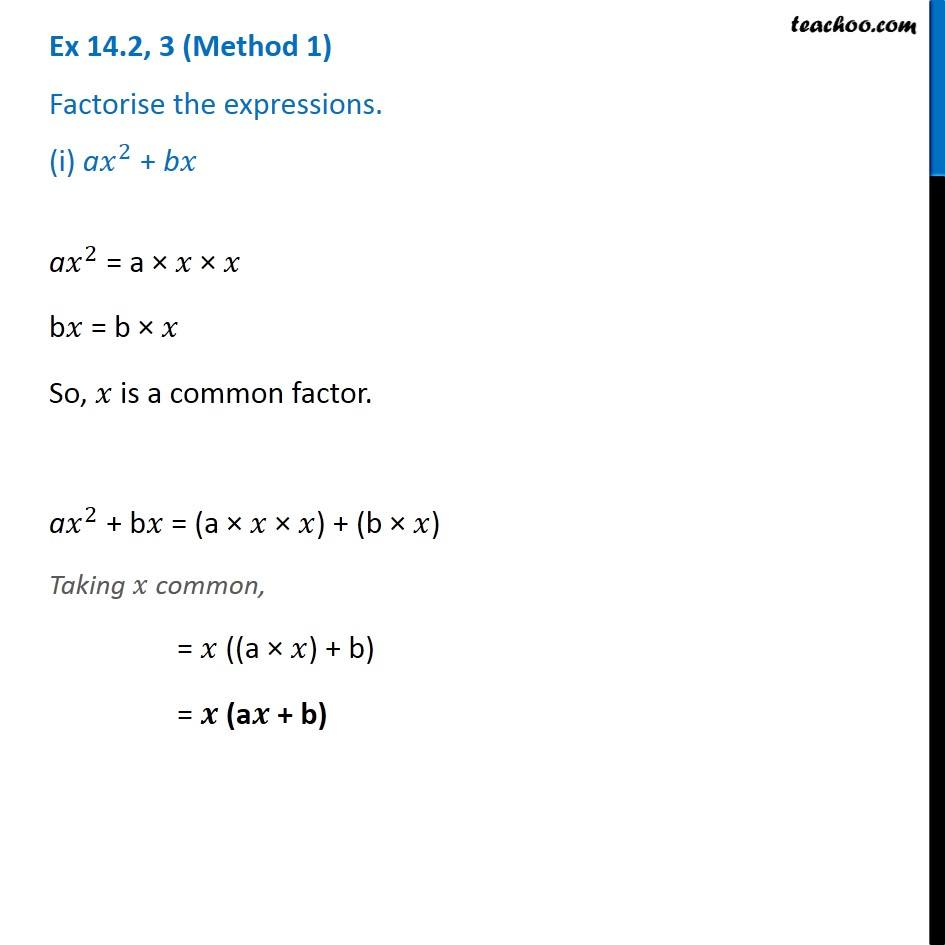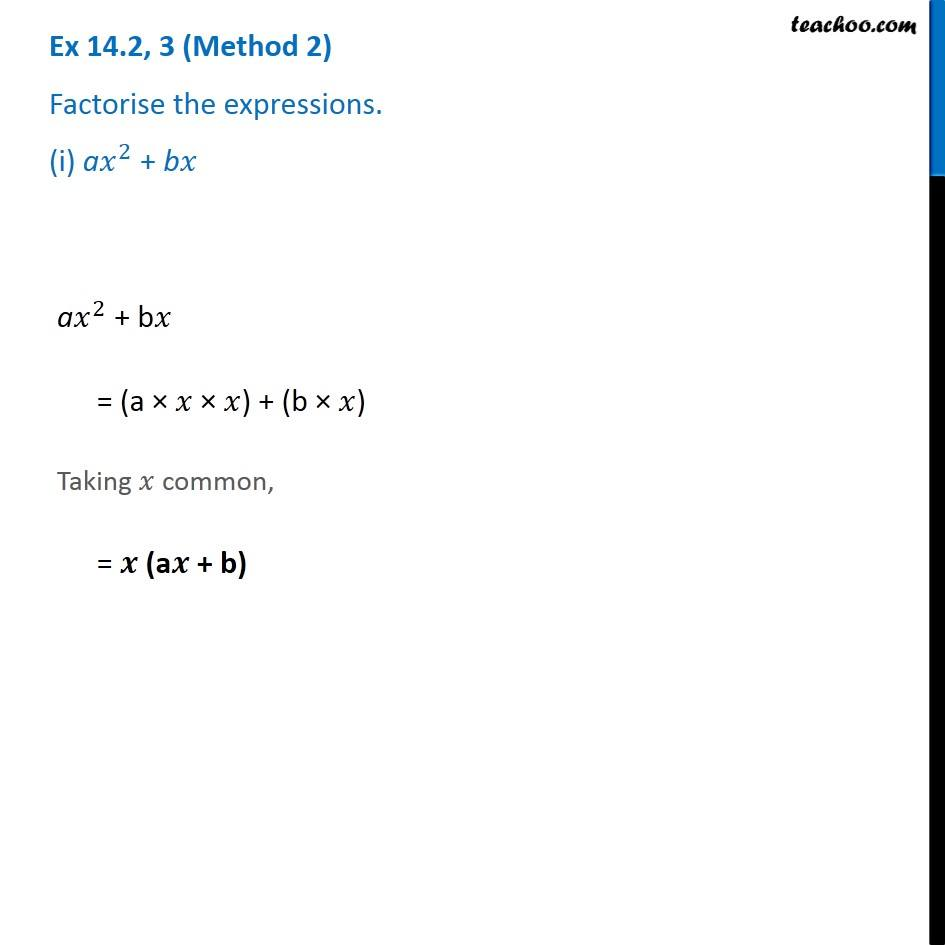1. Chapter 14 Class 8 Factorisation
2. Serial order wise
3. Ex 14.2

Transcript

Ex 14.2, 3 (Method 1) Factorise the expressions. (i) a𝑥^2 + b𝑥 a𝑥^2 = a × 𝑥 × 𝑥 b𝑥 = b × 𝑥 So, 𝑥 is a common factor. a𝑥^2 + b𝑥 = (a × 𝑥 × 𝑥) + (b × 𝑥) Taking 𝑥 common, = 𝑥 ((a × 𝑥) + b) = 𝒙 (a𝒙 + b) Ex 14.2, 3 (Method 2) Factorise the expressions. (i) a𝑥^2 + b𝑥 a𝑥^2 + b𝑥 = (a × 𝑥 × 𝑥) + (b × 𝑥) Taking 𝑥 common, = 𝒙 (a𝒙 + b)

Ex 14.2